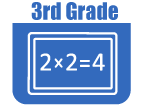Resources by Strand

Strand: MATHEMATICAL PRACTICES (3.MP)
The Standards for Mathematical Practice in Third Grade describe mathematical habits of mind that teachers should seek to develop in their students. Students become mathematically proficient in engaging with mathematical content and concepts as they learn, experience, and apply these skills and attitudes (Standards 3.MP.18).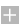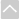Strand: OPERATIONS AND ALGEBRAIC THINKING (3.OA)
Represent and solve problems involving multiplication and division within 100 (Standards 3.OA.1–4 and Standard 3.OA.7)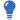Core Guide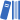OER Curriculum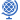Instructional TasksReview Activitiy

Standard 3.OA.1
Interpret products of whole numbers, such as interpret 5 × 7 as the total number of objects in 5 groups of 7 objects each. For example, describe a context in which a total number of objects can be expressed as 5 × 7.

Standard 3.OA.2
Interpret whole-number quotients of whole numbers. For example, interpret 56 ÷ 8 as the number of objects in each share when 56 objects are partitioned equally into eight shares (partitive), or as a number of shares when 56 objects are partitioned into equal shares of eight objects each (quotative).

Standard 3.OA.3
Use multiplication and division within 100 to solve word problems in situations involving equal groups, arrays, and measurement quantities. For example, use drawings and equations with a symbol for the unknown number to represent the problem.

Standard 3.OA.4
Determine the unknown whole number in a multiplication or division equation relating three whole numbers. For example, determine the unknown number - product, factor, quotient, dividend, or divisor - that makes the equation true in each of the equations 8 x ? = 48, 5 = ? ÷ 3, 6 x 6 = ?.

They demonstrate understanding of the properties of multiplication and the relationship between multiplication and division (Standards 3.OA.5–6)Core GuideOER CurriculumInstructional TasksReview Activitiy

Standard 3.OA.5
Apply properties of operations as strategies to multiply and divide. For example: If 6 x 4 = 24 is known, then 4 x 6 = 24 is also known (commutative property of multiplication). 3 x 5 x 2 can be found by 3 x 5 = 15, then 15 x 2 = 30, or by 5 x 2 = 10, then 3 x 10 = 30 (associative property of multiplication). Knowing that 8 x 5 = 40 and 8 x 2 = 16, one can find 8 x 7 as 8 x (5 + 2) = (8 x 5) + (8 x 2) = 40 + 16 = 56 (distributive property). (Third grade students may, but need not, use formal terms for these properties.)

Standard 3.OA.6
Understand division as an unknown-factor problem. Understand the relationship between multiplication and division (multiplication and division are inverse operations). For example, find 32 ÷ 8 by finding the number that makes 32 when multiplied by 8.

Represent and solve problems involving multiplication and division within 100 (Standards 3.OA.1–4 and Standard 3.OA.7)Core GuideOER CurriculumInstructional TasksReview Activitiy

Standard 3.OA.7
Fluently multiply and divide.

1. Fluently multiply and divide within 100, using strategies such as the relationship between multiplication and division or properties of operations. (For example, knowing that 8 x 5 = 40, one knows 40 ÷ 5 = 8).
Students use the four operations to identify and explain patterns in arithmetic (Standards 3.OA.8–9)Core GuideOER CurriculumInstructional TasksReview Activitiy

Standard 3.OA.8
Solve two-step word problems.

1. Solve two-step word problems using the four operations. Know how to perform operations in the conventional order when there are no parentheses to specify a particular order (Order of Operations). (Limit to problems posed with whole numbers and having whole number answers.)
2. Represent two-step problems using equations with a letter standing for the unknown quantity. Create accurate equations to match word problems.
3. Assess the reasonableness of answers using mental computation and estimation strategies, including rounding.

Standard 3.OA.9
Identify arithmetic patterns (including patterns in the addition table or multiplication table), and explain them using properties of operations. For example, observe that four times a number is always even, and explain why four times a number can be decomposed into two equal addends.Strand: NUMBER AND OPERATIONS IN BASE TEN (3.NBT)
Use place value understanding and properties of operations to perform multi-digit arithmetic. A range of algorithms may be used (Standards 3.NBT.1–3).Core GuideOER CurriculumInstructional TasksReview Activitiy

Standard 3.NBT.1
Use place value understanding to round whole numbers to the nearest 10 or 100.

Standard 3.NBT.2
Fluently add and subtract within 1,000 using strategies and algorithms based on place value, properties of operations, and/or the relationship between addition and subtraction.

Standard 3.NBT.3
Multiply one-digit whole numbers by multiples of 10 in the range 1090 (for example, 9 x 80 and 5 x 60) using strategies based on place value and properties of operations.Strand: NUMBER AND OPERATIONS - FRACTIONS (3.NF)
Develop understanding of fractions as numbers. Denominators are limited to 2, 3, 4, 6, and 8 in third grade. (3.NF.1-3)Review ActivitiyOER CurriculumInstructional Tasks

Standard 3.NF.1
Understand that a unit fraction has a numerator of one and a non-zero denominator.

1. Understand a fraction 1/b as the quantity formed by one part when a whole is partitioned into b equal parts.
2. Understand a fraction a/b as the quantity formed by a parts of size 1/b. For example: 1/4 + 1/4 + 1/4 = 3/4.

Standard 3.NF.2
Understand a fraction as a number on the number line; represent fractions on a number line diagram.

1. Represent a fraction 1/b on a number line diagram by defining the interval from 0 to 1 as the whole and partitioning it into b equal parts. Recognize that each part has size 1/b and that the endpoint of the part based at 0 locates the number 1/b on the number line.
2. Represent a fraction a/b on a number line diagram by marking off a lengths 1/b from 0. Recognize that the resulting interval has size a/b and that its endpoint locates the number a/b on the number line.

Standard 3.NF.3
Explain equivalence of fractions in special cases, and compare fractions by reasoning about their size.

1. Understand two fractions as equivalent if they are the same size, or the same point on a number line.
2. Recognize and generate simple equivalent fractions, such as 1/2 = 2/4, 4/6 = 2/3. Explain why the fractions are equivalent by using a visual fraction model, for example.
3. Express whole numbers as fractions, and recognize fractions that are equivalent to whole numbers. For example, express 3 in the form 3 = 3/1; recognize that 6/1 = 6; locate 4/4 and 1 at the same point of a number line diagram.
4. Compare two fractions with the same numerator or the same denominator by reasoning about their size. Recognize that comparisons are valid only when the two fractions refer to the same whole. Record the results of comparisons with the symbols >, =, or <, and justify the conclusions, for example, by using a visual fraction model.Strand: MEASUREMENT AND DATA (3.MD)
Solve problems involving measurement and estimation of intervals of time, liquid volumes, and masses of objects. (3.MD.1–2)Core GuideOER CurriculumInstructional TasksReview Activitiy

Standard 3.MD.1
Tell and write time to the nearest minute and measure time intervals in minutes. Solve word problems involving addition and subtraction of time intervals in minutes, for example, by representing the problem on a number line diagram.

Standard 3.MD.2
Measure and estimate liquid volumes and masses of objects using standard units of grams (g), kilograms (kg), milliliters (ml), and liters (l). (Excludes compound units such as cubic centimeters [cc or cm3] and finding the geometric volume of a container.) Add, subtract, multiply, or divide to solve one-step word problems involving masses of objects or volumes of liquids that are given in the same units, for example, by using drawings (such as a beaker with a measurement scale) to represent the problem. (Excludes multiplicative comparison problems.)

Represent and interpret data (Standards 3.MD.3– 4)Core GuideOER CurriculumInstructional TasksReview Activitiy

Standard 3.MD.3
Draw a scaled picture graph and a scaled bar graph to represent a data set with several categories. Solve one- and two-step "how many more" and "how many less" problems using information presented in scaled bar graphs. For example, draw a bar graph in which each square in the bar graph might represent five pets.

Standard 3.MD.4
Generate measurement data by measuring lengths using rulers marked with halves and fourths of an inch. Show the data by making a line plot where the horizontal scale is marked off in appropriate units-whole numbers, halves, or quarters.

Understand concepts of area and relate area to multiplication and addition (Standards 3.MD.5–7)Core GuideOER CurriculumInstructional TasksReview Activitiy

Standard 3.MD.5
Recognize area as an attribute of plane figures and understand concepts of area measurement.

1. A square with side length one unit, called "a unit square," is said to have "one square unit" of area, and can be used to measure area.
2. A plane figure which can be covered without gaps or overlaps by n unit squares is said to have an area of n square units.

Standard 3.MD.6
Measure area by counting unit squares (square centimeters, square meters, square inches, square feet, and improvised units).

Standard 3.MD.7
Relate area to the operations of multiplication and addition (refer to 3.OA.5).

1. Find the area of a rectangle with whole-number side lengths by tiling it, and show that the area is the same as would be found by multiplying the side lengths.
2. Multiply side lengths to find areas of rectangles with whole-number side lengths in the context of solving real-world and mathematical problems, and represent wholenumber products as rectangular areas in mathematical reasoning.
3. Use tiling to show in a concrete case that the area of a rectangle with whole-number side lengths a and b + c is the sum of a x b and a x c. Use area models to represent the distributive property in mathematical reasoning.
4. Recognize area as additive. Find areas of rectilinear figures by decomposing them into non- overlapping rectangles and adding the areas of the non-overlapping parts, applying this technique to solve real-world problems.
Recognize perimeter as an attribute of plane figures and distinguish between linear and area measures (Standard 3.MD.8)Core GuideOER CurriculumInstructional TasksReview Activitiy

Standard 3.MD.8
Solve real-world and mathematical problems involving perimeters of polygons, including finding the perimeter given the side lengths, finding an unknown side length, and exhibiting rectangles with the same perimeter and different areas or with the same area and different perimeters.Strand: GEOMETRY (3.G)
Reason with shapes and their attributes. (Standards 3.G.1–2)Core GuideOER CurriculumInstructional TasksReview Activitiy

Standard 3.G.1
Understand that shapes in different categories (for example, rhombuses, rectangles, and others) may share attributes (for example, having four sides), and that the shared attributes can define a larger category (for example, quadrilaterals). Recognize rhombuses, rectangles, and squares as examples of quadrilaterals, and draw examples of quadrilaterals that do not belong to any of these subcategories.

Standard 3.G.2
Partition shapes into parts with equal areas. Express the area of each part as a unit fraction of the whole. For example, partition a shape into four parts with equal area, and describe the area of each part as 1/4 of the area of the shape.The Online Core Resource pages are a collaborative project between the Utah State Board of Education and the Utah Education Network. If you would like to recommend a high quality resource, contact Trish French (Elementary) or Lindsey Henderson (Secondary). If you find inaccuracies or broken links contact resources@uen.org.